• 知识交点：决策树剪枝 为什么要剪枝 一颗完全生长的决策树难免会遇到过拟合的情况。因此，我们需要对决策树进行剪枝，提升模型的泛化能力。 决策树的剪枝操作通常有两种方法，预剪枝与后剪枝。 预剪枝 预剪枝的核心...

更多深度学习资讯都在公众号：深度学习视觉

为什么要剪枝 一颗完全生长的决策树难免会遇到过拟合的情况。因此，我们需要对决策树进行剪枝，提升模型的泛化能力。
决策树的剪枝操作通常有两种方法，预剪枝与后剪枝。
预剪枝 预剪枝的核心思想是在树中节点进行扩展之前，先计算当前的划分是否能带来模型泛化能力的提升，如果不能，则不再继续生长子树。此时可能存在不同类别的样本同时存于节点中，可以按照多数投票的原则判断该节点所属类别。预剪枝对于何时停止决策树的生长有以下几种方法： (1) 当树达到一定深度的时候，停止树的生长； (2) 当到达当前节点的样本数量小于某个阈值的时候，停止树的生长； (3) 计算每次分裂对测试集的准确度的提升，当小于某个阈值的时候，不再继续生长。 预剪枝具有思想直接、算法简单、效率高等特点，适合解决大规模数据的问题。但是，对于上述阈值，需要经验判断。此外，预剪枝有欠拟合的风险，因为，虽然当前的划分会导致测试集准确率降低或提升不高，但在之后的划分中，准确率可能会有显著提升。
后剪枝 后剪枝的核心思想是让算法生成一颗完全生长的决策树，然后自底向上计算是否剪枝。剪枝过程将子树删除，有一个叶子节点替代，该节点的类别同样用投票决定。同样，后剪枝也可以通过在测试集上的准确率来判断，如果剪枝过后准确率有所提升，则进行剪枝。相比于预剪枝，后剪枝的泛化能力更强，但是计算开销会更大。
后剪枝方法： 错误率降低剪枝(Reduced Error Pruning,REP)、悲观剪枝(Pessimistic Error Pruning,PEP)、代价复杂度剪枝(Cost Complexity Pruning,CCP)、最小误差剪枝(Minimum Error Pruning,MEP)、CVP(Critical Value Pruning)、OPP(Optimal Pruning)等。
CCP代价复杂度剪枝： (1) 从完整决策树

T

0

T_0

开始，生成一个子树序列

{

T

0

,

T

1

,

T

2

,

.

.

.

,

T

n

}

\{T_0,T_1,T_2,...,T_n\}

，其中

T

i

+

1

T_{i+1}

由

T

i

T_i

生成。

T

n

T_n

为树的根节点（剪到最后只剩根节点）。 (2) 在子树序列中，根据真实误差选择最佳的决策树。 详细剪枝步骤： 步骤(1)从

T

0

{T_0}

开始，剪枝

T

i

T_i

中关于训练数据集合误差增加最小的分支以得到

T

i

+

1

T_{i+1}

。具体的，当一棵树

T

T

在节点

t

t

处剪枝时，它的误差增加可以用

R

(

t

)

−

R

(

T

t

)

R(t)-R(T_t)

表示，其中

R

(

t

)

R(t)

表示进行剪枝之后的该节点误差，

R

(

T

t

)

R(T_t)

表示未进行剪枝时子树

T

t

T_t

的误差。考虑到树的复杂性因素，我们用

∣

L

(

T

t

)

∣

|L(T_t)|

表示子树

T

t

T_t

的叶子节点个数，则树在节点

t

t

处剪枝后的误差增加率为

α

=

R

(

t

)

−

R

(

T

t

)

∣

L

(

T

t

)

∣

−

1

\alpha = \frac{R(t)-R(T_t)} {|L(T_t)|-1}

在得到

T

t

T_t

后，我们每一步选择

α

\alpha

最小的节点进行剪枝。
步骤(2),我们需要从子树序列中选出真实误差最小的决策树。CCP给出了两种常用的方法：一种是基于独立剪枝数据集，该方法与REP类似，但是由于只能从子树序列

{

T

0

,

T

1

,

T

2

,

.

.

.

,

T

n

}

\{T_0,T_1,T_2,...,T_n\}

中选择最佳决策树，而非像REP能在所有可能的子树中寻求最优解，因此模型效果上会有一定的不足。另一只方案是采取K折交叉验证来选取最优决策树。
总结 代价复杂度剪枝使用交叉验证策略的时候，不需要用到测试数据集，精度与REP差不多，但形成的树复杂度小。但是，由于生成子树序列的时间复杂度与原始决策树的非叶子节点个数呈现二次关系，导致算法相比REP、PEP、MEP等线性复杂度的后剪枝方法运行时间更大。
剪枝过程在决策树模型中占据着极其重要的地位，有很多研究表明，剪枝比树的生成更为关键。对于不同划分标准生成的过拟合决策树，在经过剪枝之后都能保留最重要的属性划分，因此最终的性能差距并不大。
展开全文• 主要介绍了决策树剪枝算法的python实现方法,结合实例形式较为详细的分析了决策树剪枝算法的概念、原理并结合实例形式分析了Python相关实现技巧,需要的朋友可以参考下
• ## 决策树剪枝(cart剪枝)的原理介绍

万次阅读 多人点赞 2018-01-17 15:05:07
1.决策树剪枝决策树是一种分类器，通过ID3，C4.5和CART等算法可以通过训练数据构建一个决策树。但是，算法生成的决策树非常详细并且庞大，每个属性都被详细地加以考虑，决策树的树叶节点所覆盖的训练样本都是“纯”...
最近看《统计学习方法》，最后有一部分讲到cart树的剪枝策略，个人觉得书上讲得比较晦涩难懂，现在结合个人理解和大家分享下，如有不正，敬请谅解！
1.决策树剪枝

决策树是一种分类器，通过ID3，C4.5和CART等算法可以通过训练数据构建一个决策树。但是，算法生成的决策树非常详细并且庞大，每个属性都被详细地加以考虑，决策树的树叶节点所覆盖的训练样本都是“纯”的。因此用这个决策树来对训练样本进行分类的话，你会发现对于训练样本而言，这个树表现完好，误差率极低且能够正确得对训练样本集中的样本进行分类。训练样本中的错误数据也会被决策树学习，成为决策树的部分，但是对于测试数据的表现就没有想象的那么好，或者极差，这就是所谓的过拟合(Overfitting)问题。

决策树剪枝主要可以分为两种：
预剪枝和后剪枝

预剪枝（Pre-Pruning）
在构造决策树的同时进行剪枝。所有决策树的构建方法，都是在无法进一步降低熵的情况下才会停止创建分支的过程，为了避免过拟合，可以设定一个阈值，熵减小的数量小于这个阈值，即使还可以继续降低熵，也停止继续创建分支。但是这种方法实际中的效果并不好，因为在实际中，面对不同问题，很难说有一个明确的阈值可以保证树模型足够好，当然在xgboost和lightGBM里面有一些参数例如min_child_weight也算是设置了分裂节点的权重值，像xgboost之类已经把过拟合写进损失函数中了，因此不需要有剪枝的过程。
后剪枝（Post-Pruning）
后剪枝的剪枝过程是删除一些子树，然后用其叶子节点代替，在剪枝过程中, 将一些子树删除而用叶节点代替,这个叶节点所标识的类别用这棵子树中大多数训练样本所属的类别来标识。
决策树构造完成后进行剪枝。剪枝的过程是对拥有同样父节点的一组节点进行检查，判断如果将其合并，熵的增加量是否小于某一阈值。如果确实小，则这一组节点可以合并一个节点，其中包含了所有可能的结果。后剪枝是目前最普遍的做法。
剪枝作为决策树后期处理的重要步骤，是必不可少的。没有剪枝，就是一个完全生长的决策树，是过拟合的，需要去掉一些不必要的节点以使得决策树模型更具有泛化能力。

2.CART剪枝

树上的流程基本是这样：

对于原始的CART树A0，先剪去一棵子树，生成子树A1，然后再从A1剪去一棵子树生成A2，直到最后剪到只剩一个根结点的子树An，于是得到了A0-AN一共n+1棵子树。

然后再用n+1棵子树预测独立的验证数据集，谁的误差最小就选谁，大致的思路就是这样。

然后需要怎么去生成这些树呢，每棵树根据什么取选择剪枝，书上我一直看不太懂是下面这个：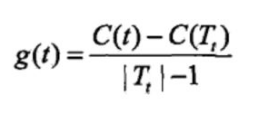书里对g(t)的解释是：它表示剪枝后整体损失函数减少的程度。。。，然而我当时并不是很理解，这么说应该剪掉以g(t)最大结点为根的子树，因为g(t)最大，那么剪枝后整体损失

函数减少程度也最大。但书中的算法却说优先剪去g(t)最小的子树，这就不懂为啥要从小开始剪枝，后来上网查了之后才懂。

实际上这个g(t)表示剪枝的阈值，即对于某一结点a，当总体损失函数中的参数alpha = g(t)时，剪和不剪总体损失函数是一样的（这可以在书中（5.27）和（5.28）联立得到）。这时如果alpha稍稍增大，那么不剪的整体损失函数就大于剪去的。即alpha大于g(t)该剪，剪了会使整体损失函数减小；alpha小于g(t)不该剪，剪了会使整体损失函数增大。（请注意上文中的总体损失函数，对象可以是以a为根的子树，也可以是整个CART树，对a剪枝前后二者的总体损失函数增减是相同的。）对于同一棵树的结点，alpha都是一样的，当alpha从0开始缓慢增大，总会有某棵子树该剪，其他子树不该剪的情况，即alpha超过了某个结点的g(t)，但还没有超过其他结点的g(t)。这样随着alpha不断增大，不断地剪枝，就得到了n+1棵子树，接下来只要用独立数据集测试这n+1棵子树，试试哪棵子树的误差最小就知道那棵是最好的方案了。
By the way，书上第二版的最后貌似迭代过程是错的，应该是回到步骤2, 因为，每次都需要逐步增加g(t)，这样遍历所有的g(t)之后就一定会剪成一颗只有一个根节点的树，这个大家可以好好理解。这里有张图，说明为什么要选择小的g(t)值，看看应该就能懂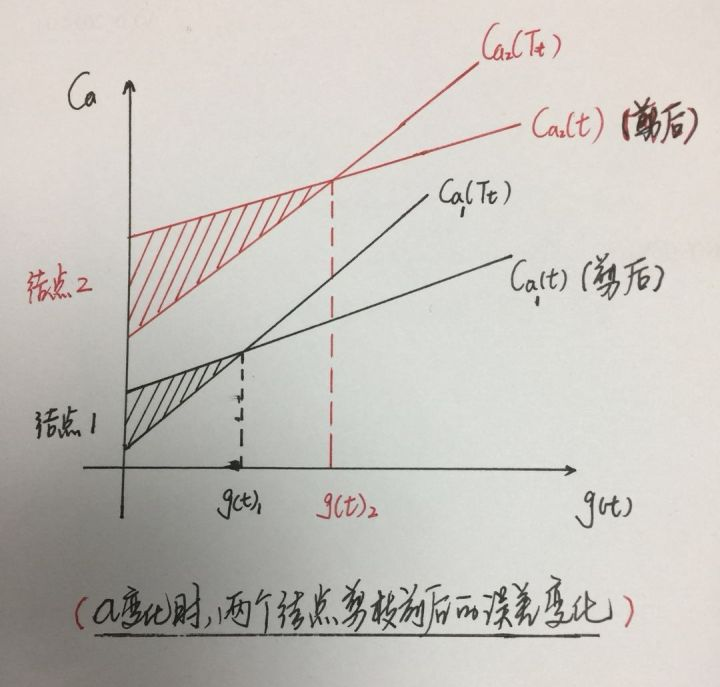为什么要选择最小的g(t)呢？以图中两个点为例，结点1和结点2，g(t)2大于g(t)1, 假设在所有结点中g(t)1最小，g(t)2最大，两种选择方法：当选择最大值g(t)2，即结点2进行剪枝，但此时结点1的不修剪的误差大于修剪之后的误差，即如果不修剪的话，误差变大，依次类推，对其它所有的结点的g(t)都是如此，从而造成整体的累计误差更大。反之，如果选择最小值g(t)1，即结点1进行剪枝，则其余结点不剪的误差要小于剪后的误差，不修剪为好，且整体的误差最小。从而以最小g(t)剪枝获得的子树是该alpha值下的最优子树！
这下该明白了吧，反正我是明白了。。。有不懂可以留言
最后附上cart剪枝的正确流程图：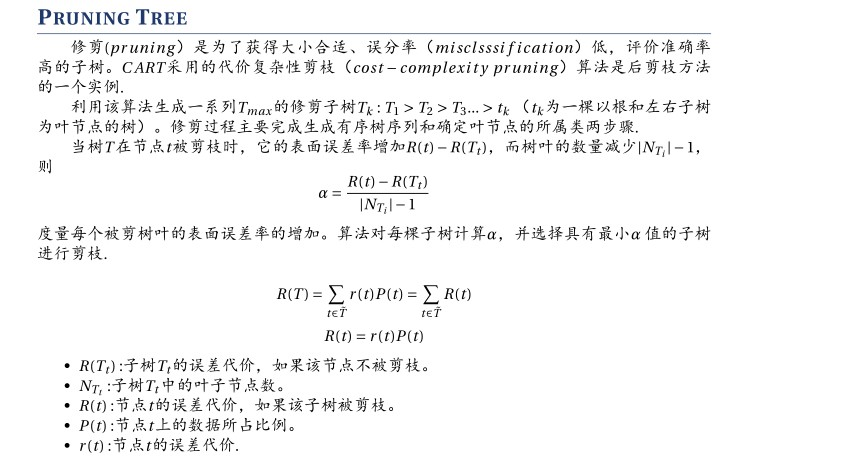展开全文机器学习
• CART剪枝以及决策树剪枝中α相关问题CART剪枝过程问题1：$g(t)$的含义：问题2：为什么选择$g(t)$最小的$T_t$进行剪枝？问题3：剪枝过程中选取的$α$为什么一定是递增的？问题4：为什么CART剪枝过程中的子树序列\$T_1,...
CART剪枝过程
CART的剪枝过程主要分为两部分，第一部分是剪枝，形成一个子树序列；第二部分是对剪枝得到的子树序列进行交叉验证，选取最优子树。第二部分容易理解，问题在于第一部分如何剪枝。首先介绍如何剪枝，再对其中的细节进行理解。 剪枝过程中所用的损失函数为：

C

α

(

T

)

=

C

(

T

)

+

α

∣

T

∣

C_\alpha(T) = C(T)+\alpha|T|

其中，

T

T

为任意子树，

C

(

T

)

C(T)

为对训练数据的预测误差（如基尼指数、熵等），

∣

T

∣

|T|

为子树的叶结点个数，

α

≥

0

α≥0

为参数，

C

α

(

T

)

C_\alpha(T)

为参数是α时的子树T的整体损失。 从整体树

T

0

T_0

开始剪枝，对

T

0

T_0

的任意内部结点

t

t

，以

t

t

为单结点树的损失函数为

C

α

(

t

)

=

C

(

t

)

+

α

C_α (t)=C(t)+α

以

t

t

为根结点的子树

T

t

T_t

的损失函数为

C

α

(

T

t

)

=

C

(

T

t

)

+

α

∣

T

t

∣

C_α (T_t )=C(T_t )+α|T_t |

显然，在决策树中，对结点t进一步划分会降低误差，也就是

C

(

t

)

>

C

(

T

t

)

C(t)>C(T_t )

而由于划分使得

∣

T

t

∣

>

1

|T_t |>1

所以存在某一

α

α

有

C

α

(

T

t

)

=

C

α

(

t

)

C_α (T_t )=C_α (t)

此时

α

=

C

(

t

)

−

C

(

T

t

)

∣

T

t

∣

−

1

α=\frac{C(t)-C(T_t )}{|T_t |-1}

所以对于每一个内部结点

t

t

都有这样一个

α

α

，令其为

g

(

t

)

=

C

(

t

)

−

C

(

T

t

)

∣

T

t

∣

−

1

g(t)=\frac{C(t)-C(T_t )}{|T_t |-1}

从

T

0

T_0

中选择

g

(

t

)

g(t)

最小的

T

t

T_t

进行剪枝，

g

(

t

)

g(t)

记为

α

1

α_1

，得到新的子树作为

T

1

T_1

，再在

T

1

T_1

上计算所有内部结点的

g

(

t

)

g(t)

，重复上述过程，直到所得子树只有根结点和两个叶结点构成，过程中产生的子树序列

T

1

,

T

2

,

…

,

T

n

T_1,T_2,…,T_n

对应参数序列

α

1

,

α

2

,

…

,

α

n

α_1,α_2,…,α_n

。
Q1：

g

(

t

)

g(t)

的含义：

C

(

t

)

−

C

(

T

t

)

C(t)-C(T_t )

可以理解为在构建决策树的过程中，将单结点t进一步划分后训练数据上的误差下降量，

∣

T

t

∣

−

1

|T_t |-1

则是进一步划分后决策树增加的结点数。因此，

g

(

t

)

g(t)

可以理解为将单结点t进一步划分后，平均每个结点带来的误差下降量，即每个结点的误差减小率。
Q2：为什么选择

g

(

t

)

g(t)

最小的

T

t

T_t

进行剪枝？
上一个问题中知道了

g

(

t

)

g(t)

的含义，另一方面，剪枝的目的在于减小决策树对训练数据的拟合程度，防止过拟合。因此，在尽量简化决策树的条件下，选择每个结点带来的误差下降量最少的子树进行剪枝显然是一个明智之举。也就是说，这个子树带来的“价值”弥补不了带来的“危害”，不如不要。
Q3：剪枝过程中选取的

α

α

为什么一定是递增的？
《统计学习方法》P72说

0

<

α

1

<

α

2

<

⋯

<

α

n

<

∞

0<α_1<α_2<⋯<α_n<∞

，而剪枝过程中只选取每一轮算出的最小

g

(

t

)

g(t)

（即

α

α

），为何依次选取的

α

α

一定是递增的呢？这分两种情况：
后一轮选取的剪枝点与前一轮选取剪枝点不在同一支上，则前一轮剪枝不会影响后一轮选中点的

g

(

t

)

g(t)

（即

α

α

），该值显然不小于前一轮剪枝点的

g

(

t

)

g(t)

（即

α

α

）。如图：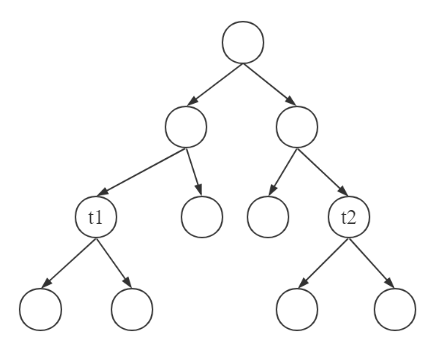后一轮选取的剪枝点与前一轮选取的剪枝点在同一支上，如图：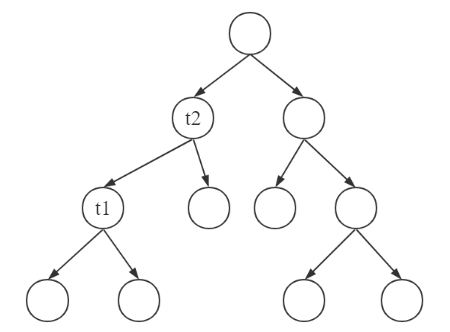则第一轮有：（上标表示轮次）

g

1

(

t

1

)

=

C

(

t

1

)

−

C

(

T

t

1

)

∣

T

t

1

∣

−

1

g^1 (t_1)=\frac{C(t_1 )-C(T_{t_1})}{|T_{t_1} |-1}

g

1

(

t

2

)

=

C

(

t

2

)

−

C

(

T

t

2

1

)

∣

T

t

2

1

∣

−

1

g^1 (t_2 )=\frac{C(t_2 )-C(T_{t_2}^1 )}{|T_{t_2}^1|-1}

第二轮有

g

2

(

t

2

)

=

(

C

(

t

2

)

−

C

(

T

t

2

2

)

)

(

∣

T

t

2

2

∣

−

1

)

g^2 (t_2)=\frac{(C(t_2 )-C(T_{t_2}^2 ))}{(|T_{t_2}^2 |-1)}

第一轮的剪枝给第二轮选中点带来的影响可以表示为：

∣

T

t

2

2

∣

=

∣

T

t

2

1

∣

−

∣

T

t

1

∣

+

1

|T_{t_2}^2 |=|T_{t_2}^1 |-|T_{t_1} |+1

C

(

T

t

2

2

)

=

C

(

T

t

2

1

)

+

(

C

(

t

1

)

−

C

(

T

t

1

)

)

C(T_{t_2}^2 )= C(T_{t_2}^1 )+(C(t_1 )-C(T_{t_1} ))

即第一轮剪枝使得以

t

2

t_2

为根结点的子树的结点树减少，误差增大。（这很好理解，就是决策树构建的逆过程） 代入得

g

2

(

t

2

)

=

(

C

(

t

2

)

−

C

(

T

t

2

1

)

)

−

(

C

(

t

1

)

−

C

(

T

t

1

)

)

(

∣

T

t

2

1

∣

−

1

)

−

(

∣

T

t

1

∣

−

1

)

g^2 (t_2)=\frac{(C(t_2 )-C(T_{t_2}^1))-(C(t_1 )-C(T_{t_1} ))}{(|T_{t_2}^1 |-1)-(|T_{t_1} |-1)}

可以看出分子和分母的前一部分分别是

g

1

(

t

2

)

g^1 (t_2 )

的分子和分母，后一部分分别是

g

1

(

t

1

)

g^1 (t_1 )

的分子和分母，因为

g

1

(

t

2

)

>

g

1

(

t

1

)

g^1 (t_2 )>g^1 (t_1 )

，所以

g

2

(

t

2

)

>

g

1

(

t

2

)

>

g

1

(

t

1

)

g^2 (t_2 )>g^1 (t_2 )>g^1 (t_1 )

（这里可以通过转换为简单的代数问题进行证明，即：假设

e

=

b

a

≥

c

d

=

f

e=\frac ba ≥ \frac cd=f

要证明

b

−

c

a

−

d

≥

f

\frac{b-c}{a-d}≥f

证：

b

−

c

a

−

d

=

a

e

−

d

f

a

−

d

≥

a

e

−

d

e

a

−

d

=

e

≥

f

\frac {b-c}{a-d}=\frac {ae-df}{a-d}≥\frac {ae-de}{a-d}=e≥f

） 所以，第二轮选取的剪枝点的

g

(

t

)

g(t)

（即

α

α

）不小于第一轮选中的剪枝点的

g

(

t

)

g(t)

（即α）。
Q4：为什么CART剪枝过程中的子树序列

T

1

,

T

2

,

…

,

T

n

T_1,T_2,…,T_n

分别是区间

α

∈

[

α

i

,

α

(

i

+

1

)

)

α∈[α_i , α_{(i+1)} )

的最优子树？
对于区间

α

∈

[

α

i

,

α

i

+

1

)

α∈[α_i ,α_{i+1})

，与当前子树不同有两种情况：
若剪去了

α

≥

α

i

+

1

α≥α_{i+1}

的子树

T

t

T_t

，有

g

(

t

)

=

C

(

t

)

−

C

(

T

t

)

∣

T

t

∣

−

1

>

α

i

g(t)=\frac{C(t)-C(T_t )}{|T_t |-1}>α_i

即

C

(

t

)

−

C

(

T

t

)

>

α

i

(

∣

T

t

∣

−

1

)

C(t)-C(T_t )>α_i (|T_t |-1)

该子树的划分降低的误差量，大于增加结点数带来的损失增量，换句话说就是，这个子树值得保留，剪掉的话会使总体误差增大（这里总体误差公式中的

α

∈

[

α

i

,

α

i

+

1

α∈[α_i , α_{i+1}

)）。若没有剪去了α<α_i的子树T_t，有

g

(

t

)

=

C

(

t

)

−

C

(

T

t

)

∣

T

t

∣

−

1

<

α

i

g(t)=\frac{C(t)-C(T_t )}{|T_t |-1}<α_i

即

C

(

t

)

−

C

(

T

t

)

<

α

i

(

∣

T

t

∣

−

1

)

C(t)-C(T_t )<α_i (|T_t |-1)

该子树的划分降低的误差量，小于增加结点数带来的损失增量，换句话说就是，这个子树不值得保留，不剪掉的话会使总体误差增大（这里总体误差公式中的

α

∈

[

α

i

,

α

i

+

1

)

α∈[α_i , α_{i+1})

）。
综上，使用

α

∈

[

α

i

,

α

i

+

1

)

α∈[α_i , α_{i+1} )

剪枝得到的子树

T

i

T_i

是该区域对应的最优子树。
Q5：

α

α

在一般决策树剪枝过程中的意义
在一般的决策树剪枝过程中，

α

α

是给定的参数，可以认为是自下而上，将平均结点降低误差小于

α

α

的子树剪去。
展开全文机器学习
• 决策树剪枝
前言
无论是分类树还是回归树，剪枝过程很重要，剪枝处理不当或是没有剪枝操作，决策树模型都是失败的，通过剪枝，可以大大提高模型准确度，避免决策树的过拟合。
C4.5剪枝
决策树生成算法递归地生成决策树，直到不能继续下去为止，产生的树对于训练集会有很好的模型准确率，但是对于未知的测试数据分类却没有那么准确，因此，对书的剪枝很重要。
决策树剪枝的算法流程如下：
输入：生成算法产生的整个树T，参数alpha
输出：修剪后的子数T-alpha
（1）计算每个节点的经验熵
（2）递归地从树的叶节点向上回缩，设回缩前后的树分别为T-B和T-A，对应的损失函数分别为C-alpha（T-B）和C-alpha（T-A），如果C-alpha（T-B）<= C-alpha（T-A），进行剪枝，将父节点变为叶子节点
（3）返回（2），直至不能剪枝为止，得到损失函数最小的子树T-alpha
CART剪枝
展开全文机器学习
• 决策树剪枝 决策树为了防止过拟合，会采用剪枝的方式，符合奥卡姆剃刀原理。决策树剪枝分为预剪枝（pre-pruning）和后剪枝（post-pruning）。 预剪枝 预剪枝是在决策树生成时限制树的生长，防止树过度生长而导致...
• 决策剪枝通常有两种方法，预剪枝（ Pre-Pruning ）和后剪枝( Post-Pruning ）。 预剪枝（ Pre-Pruning ） 预剪枝 ， 即在生成决策的过程中提前停止的增长。核心思想是在中结点进行扩展之前，先计算当前的...机器学习 算法 人工智能
• 今天看到决策树剪枝的部分，书、博客都参考了一些，发现书上有些省去的地方，而大部分博客写的又晦涩难懂，因此决定写一篇容易理解的关于决策树剪枝的文章。 本文主要依据周志华的《机器学习》和李航的《统计学习...机器学习
• 为在决策树剪枝中正确选择剪枝方法，基于理论分析和算例详细地比较了当前主要的4种剪枝方法的 计算复杂性、剪枝方式、误差估计和理论基础．与PEP相比，MEP产生的树精度较小且树较大；REP是最简单的 剪枝方法之一，但...
• CART（分类回归树）之回归树总结与树剪枝总结 CART（Classification And Regression Trees，分类回归树）与分类算法中决策树ID3算法最大的不同点在于，决策树是一种贪心算法，其要在给定时间内做出最佳选择，但并不...
• @CART决策树剪枝个人理解 本文是针对CART树的个人理解 在看统计学习方法关于CART树的剪枝是，感觉书上讲得很迷惑，因此基于其他博客以及书上内容得出自己的理解。 首先确定CART树的损失函数：C∂(T) = C(T)+∂|T|；...
• 1.决策树剪枝 决策树是一种分类器，通过ID3，C4.5和CART等算法可以通过训练数据构建一个决策树。但是，算法生成的决策树非常详细并且庞大，每个属性都被详细地加以考虑，决策树的树叶节点所覆盖的训练样本都是“纯”...
• 采用局部点云和BP神经网络的苹果树剪枝决策系统构建.pdf
• ## C4.5 树剪枝

千次阅读 2015-03-31 15:48:09
树剪枝  在决策树的创建时，由于数据中的噪声和离群点，许多分枝反映的是训练数据中的异常。剪枝方法是用来处理这种过分拟合数据的问题。通常剪枝方法都是使用统计度量，剪去最不可靠的分枝。  剪枝一般分两种...
• 文章目录CART算法1. CART生成算法①. CART回归树的生成②. CART分类树的生成2. CART剪枝算法 CART算法 CART假设决策树是二叉树，内部结点特征的取值为“是”和“否”。...（2）决策树剪枝：用验证数据集对已生成的树...
• 在《统计学习方法法》中已经提到了决策树的剪枝算法了，理所当然，我们是顺着书中提到的思路来理解下决策树剪枝的关键步骤。我们定义了 该定义表示了决策树的损失函数。whaterver它是什么，现在有了损失函数这个...机器学习 统计学
• 《机器学习实战》9.2树回归之树剪枝（tree pruning） 搜索微信公众号:‘AI-ming3526’或者’计算机视觉这件小事’ 获取更多人工智能、机器学习干货 csdn：https://blog.csdn.net/baidu_31657889/ github：...机器学习 机器学习实战
• 决策树剪枝算法1、算法目的2、算法基本思路：3、决策树损失函数4、剪枝类型：4.1 预剪枝4.2 后剪枝4.3 两种剪枝策略对比 1、算法目的 决策树的剪枝是为了简化决策树模型，避免过拟合。 同样层数的决策树，叶结点的...python
• 算法目的：决策剪枝是为了简化决策模型，避免过拟合。 同样层数的决策，叶结点的个数越多就越复杂；同样的叶结点个数的决策，层数越多越复杂。 剪枝前相比于剪枝后，叶结点个数和层数只能更多或者其中一...机器学习
• 1.决策树剪枝 首先剪枝（pruning）的目的是为了避免决策树模型的过拟合。因为决策树算法在学习的过程中为了尽可能的正确的分类训练样本，不停地对结点进行划分，因此这会导致整棵树的分支过多，也就导致了过拟合。......